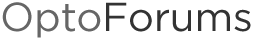# Linear Interpolation

This subroutine interpolates values using data in passed tables. This is used for calculations that must be made using values in tables that cannot easily be represented as a mathematical function. For example, I use this to calculate things like stream flow and reservoir capacity from USGS rating tables. In the subroutine, you supply the “X” table and the “Y” table with ordered data with the value in the X table corresponding to the value in the Y table at the same index. You pass an X value to the subroutine, and it interpolates a Y value.

Hi:
I am unable to open this zip archive. Can you e-,ail it to kmiller@fce.com?
Thanks

Try using IE9 (or using some other browser like Firefox). Were you using Internet Explorer version 8 when you had this trouble? That seems to be he common thread for those who’ve had trouble downloading/opening the attached zip archives.
Thanks,
-OptoMary

Hi All,

LI9_3basic.zip (1.87 KB)

I’ve attached a different format, a 9.3 BASIC chart you can import. Also, Carl’s code & comments included below.

His comment:

Linearly interpolates a value from table data. The data in the x table must be in order smallest to largest. It works best if the table sizes are exact or close to the amount of data you have.
First block: “Binary Search” OptoScript:

``````
// Find which values in the x table the passed x value falls between
// uses a binary search algorithm to constantly divide the remaining
// table in half - this is faster than the brute force method of
// starting at the beginning and moving one forward each check.

Low_Index = 0;
High_Index = Min(GetLengthOfTable(X_Table), GetLengthOfTable(Y_Table)) - 1;

repeat
Mid_Index = (High_Index + Low_Index) / 2;

if ((X_Value <= X_Table[Mid_Index]) or (X_Value  == 0.0)) then
High_Index = Mid_Index;
else
Low_Index = Mid_Index;
endif

until ((High_Index - Low_Index) <= 1);
``````

Second block “Interpolate” OptoScript:

``````low_x = x_table[low_index];
low_y = y_table[low_index];

x_range = x_table[high_index] - low_x;
y_range = y_table[high_index] - low_y;

// simple interpolation based on the values from the tables
interpolated_y = (((x_value - low_x) / max(x_range, 0.01)) * y_range) + low_y;

``````

Happy interpolating!

-OptoMary

Thanks, this subroutine is very helpful. FWIW, I used IE 11, and it wouldn’t download the zip cleanly. It saved it as attachment.php, but was fine after I manually changed the extension to .zip